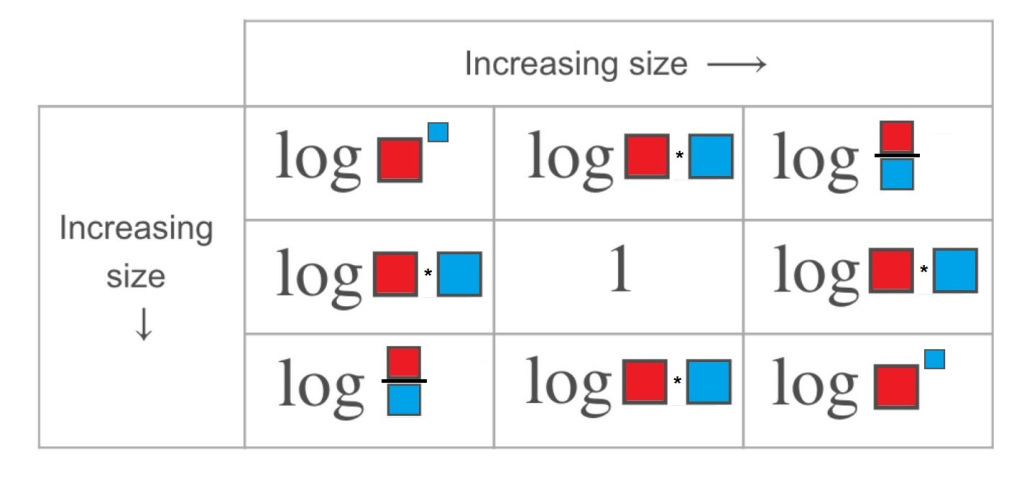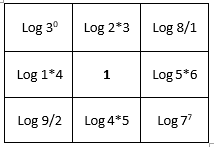# Laws of Logarithms

Directions: Using the integers 0 to 9, fill in the red and blue boxes so that the chart is accurate. You can only use a number once per red box and once per blue box. (Logs are in base 10)### Hint

What mathematical equation can be produced from a logarithm? How does that relate to the size of a logarithm?
How can you rewrite the log of a product, quotient, or power?

There are many possible solutions. One example would be:One thing to watch is the use of 0, many students will not recognize that Log 0 is undefined

Source: Bryan Anderson

## Asymptotes of Rational Functions

Directions: Using the digits 1 to 9 at most one time each, place a digit …

1.Another thing to look out for is how students interpret logx^y.
A question I had from my students was, “Do we represent log7^7 as log(7^7) or log(7)^7?”

A really great question and talking point!

2.Laws of Logarithms
Great idea right before a holiday or for a sub day.

3.What mathematical equation can be produced from a logarithm? How does that relate to the size of a logarithm?